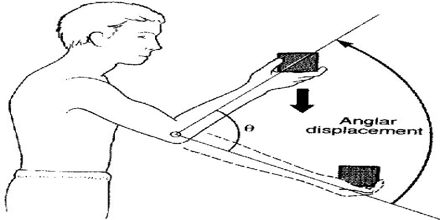Physics

# Motion of Objects Projected at an AngleThe aim of this lecture is to present and explain on Motion of Objects Projected at an Angle. here briefly describe what is the trajectory; what is the total time of the motion and what is the horizontal range. Tendency of an object to resist changes in its velocity. An object at rest has zero velocity – and (in the absence of an unbalanced force) will remain with a zero velocity. Such an object will not change its state of motion (i.e., velocity) unless acted upon by an unbalanced force.